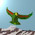## Thursday, October 21, 2010

### Theory Update 11

Recall that back in July we were looking at Koide fits for the quark masses, and it was noted that the quark equivalent of $r = \sqrt{2}$ (the parameter for the leptons) is probably around $1.76$. Today, in a comment on the previous post, someone called Dave (who I hope will soon better identify himself) has shown us (see diagram below) how simple geometry predicts a value of

$r = 1.7602838$

since the leptons lie on a circle with equation $x^2 + y^2 = r^2$ and so do the (up) quarks, and then we inscribe the blue lepton triangle inside the red quark triangle. The lepton triangle side has length $2.4494987 = \sqrt{6}$, while the quark triangle side has length $3.048901024$. This now allows us to make accurate predictions for the quark masses!1.Not to mention the CKM matrix ... recall that the (offset by 1) Koide eigenvalues could be expressed in terms of a cosine and sine, when we wrote them out with mixing matrix coefficients. So that is what these $x$ and $y$ parameters are about.

Ok, now there must be several billion people on the planet who could figure this out ... except maybe the string theorists.

2.So plugging this $1.7603$ into the Koide formula, and choosing a suitable scale, we get the masses:

1. up quarks, at a phase of $2/27$:
$17159.144$, $0.202$, $124.905$

2. down quarks, at a phase of $4/27$:
$4282.462$, $5.201$, $71.639$

3.Note that for both leptons and quarks, the triangle side length $L$ obeys

$(L/r)^2 = 3$

4.So the bigger triangle is completely defined by the ansatz of phases: sending the $2/9$ for leptons to $2/27$ for the quarks. Presumably we could keep dividing angles by $3$ and come up with an infinite sequence of Koide eigenvalue triplets this way, with the $r$ factor always determined by inscribing.

5.Good to have a clear prediction. Wikipedia gives the recent bounds on masses (see this). The most recent estimates for u and d quark masses are 5 and 20 MeV.

I assume that the order of masses is t,u, c and b,d, s and unit is GeV.

For d the prediction would be at the lower boundary of 2-15 MeV range. For s 71.639 MeV is below 100-130 MeV. For b prediction would be in the required range.

For U type quarks the predictions would be by order of magnitude too small as compared to the lower bounds.

6.Using the known relationship:

Mt/sqrt2=(cosOw+sinOw)Mz
(Ow is Wienberg angle)

to scale the Top mass, we have:

2.05MeV 1.27GeV 174.55GeV

7.Cool, Dave. Yes, I was a bit rough with the scale choice above. And I think the down quark radius should be reselected from the phase choice. It was the down quarks that did not quite fit the 1.76 before.

8.Actually, no, the down quarks work best at $1.76$, which comes from the phase $\theta = 2/27$ in the relation

$r = \sqrt{2} \sin (5 \pi/6 - 2/9 + \theta) / \sin (\pi/6)$

Then the best Koide fits give us:

1. up quarks at $\mu = 22990$,
$174.553$ GeV, $1.271$ GeV, $2.054$ MeV

2. down quarks at $\theta = 4/27$, $\mu = 635$,
$4771$ MeV, $79.8$ MeV, $5.8$ MeV

and the down quark set really pushes the experimental limits, as before.

9.... or maybe we should try to put the down quarks on the big triangle?

10.One other possibility for the down quarks is to set $r$ at the average of $r(2/27) = 1.76$ and $r(2/9) = \sqrt{2}$. Then at $\mu = 710$ and $\theta = 5/54$ the triplet is:

$4728$ MeV, $80.57$ MeV, $4.85$ MeV

At least the experimental bounds really are testing these different scenarios.

11.compare to the mass of top quark.

12.Oh, I didn't bother actually looking up the latest top quark estimates. I'm sure the experimentalists can do that, and fix up the Koide fits if necessary.

13.So according to PDG, we just need to down scale $\mu$ a little bit.

14.Actually, who was the first one suggesting the triangle presentation of Koide's angles, and in which paper or blog entry? I guess it was Carl, but I am not sure, it is the first time I see it.

15.Ok, it is http://www.brannenworks.com/koidehadrons.pdf formula 46

16.Ok, I see what puzzles me of the triangle interpretation: that there is not a clear role for the sqrt(2) in the radius of the circle, while in Foot's interpretation is the mark of an angle of 45 degrees with the unbroken "halfmass vector".

17.Alejandro, I believe it was Hans de Vries who first pointed the triangle out, some years ago. And yes, there are many ways to look at the $\sqrt{2}$ geometrically ... but two dimensions at a time, please!

18.After some thought, yes, it could be that the 45 degrees angle is a redherring. The "angle with 1,1,1", sqrt(2/3), has really two different pieces: the sqrt(3) comes from the number of families, and the sqrt(2), is the size of the symmetry breaking, as it appears very naturally in eq 46 of Brannen's.

19.Oh, and in the mixing matrix, the parameter $1$ gives an angle of 45 degrees. The $\sqrt{2}$ instead gives an angle of $0.615$ radians, whatever that means.

20.Ah, the $0.615$ seems to come from the inverse sine of $1/ \sqrt{3}$. That links the $2$ and the $3$ then.

21.That is, the number of generations ($= 3$) gives a sine value, and if we use this angle with a tan function (like in my mixing matrices) then it gives the $\sqrt{2}$.

Note: Only a member of this blog may post a comment.